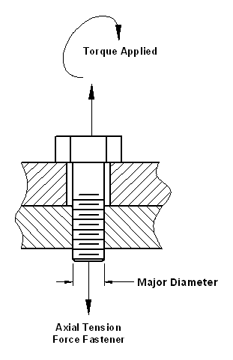#### Estimated Fastener Bolt Clamp Force Torque CalculatorSee Torque Force Design Equations webpage and torque chart page for recommended fastener torque and equation data.

This fastener bolt torque calculator will Estimate the unknown torque, diameter, axial force applied and coefficient of turning friction for the given conditions. Minimum three (3) inputs are required then select "Find" button to get unknown

This Calculator Requires a JAVA enabled browser to function properly.

Instructions:

1) Enter known number values (two inputs minimum) within center located input boxes.

2) Select associated lower button to calculate unknown value.

Note: This calculator initially assumes dry and perfect threads made from steel and/or zinc plated. Calculated values are approximate. Calculated values do not compensate for materials stiffness.

Bolt Torque Force Design Equations

 Torque Applied (N-mm, in-lbs) = Fastener Bolt Major Diameter (mm, in.) = Coefficient of Friction Steel 0.2 Cadmium 0.16 Lubricated 0.16 - 0.17 = Axial Bolt "Clamp" Force (N, lbs) =

Related: